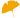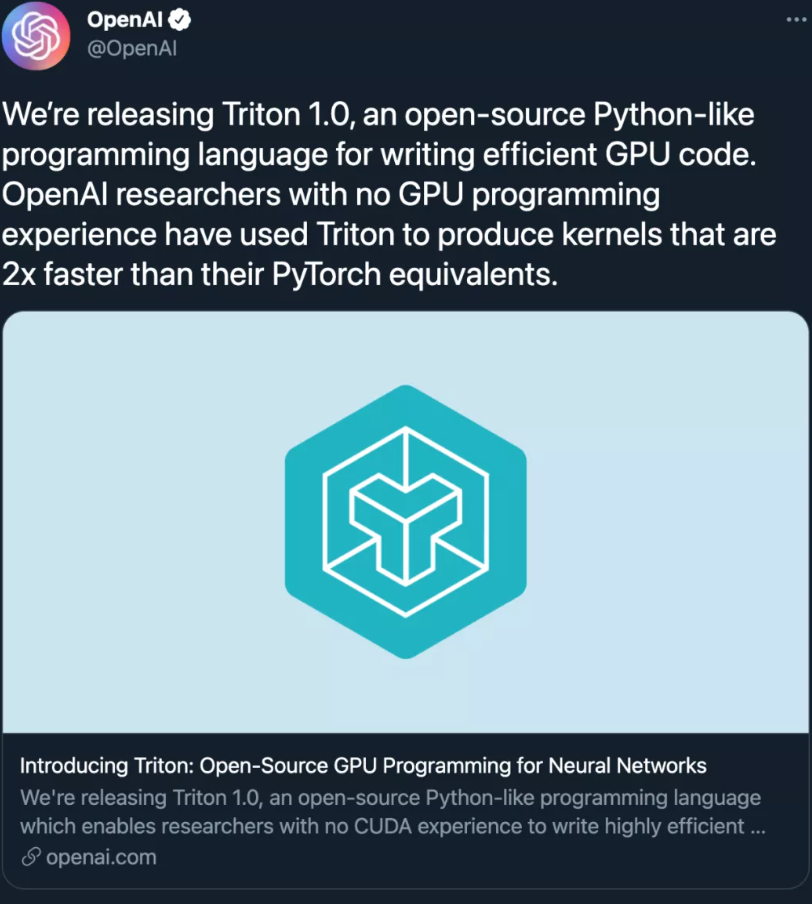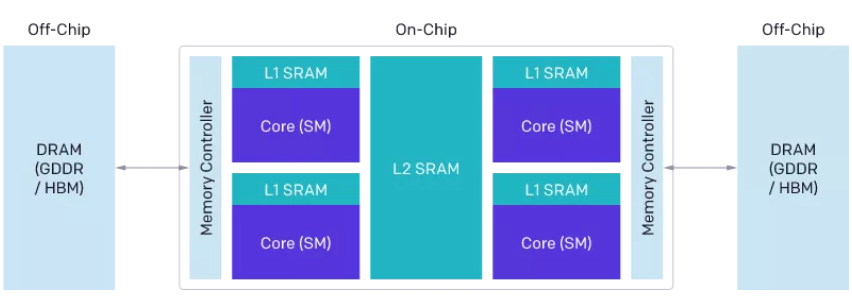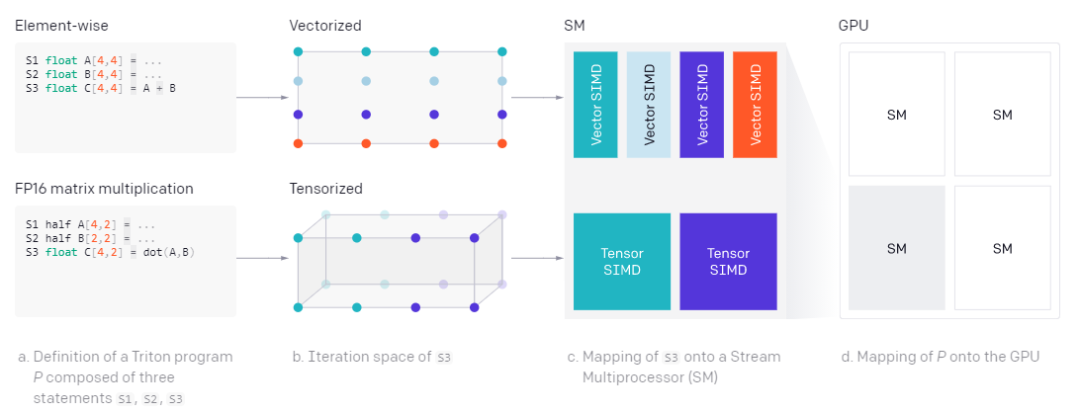### 【刚刚开源！】超级优秀地解决Python人工智能计算慢问题(附源码+长期更新+必会)OpenAI 开源了全新的 GPU 编程语言 Triton，它能成为 CUDA 的替代品吗？Tillet 希望解决的问题是打造一种比英伟达的 CUDA 等特定供应商库更好用的库，能够处理神经网络中涉及矩阵的各种操作，具备可移植性，且性能可与 cuDNN 或类似的供应商库相媲美。团队表示：「直接用 CUDA 进行 GPU 编程太难了，比如为 GPU 编写原生内核或函数这件事，会因为 GPU 编程的复杂性而出奇困难。」GPU 编程面临的挑战（重点）

• 来自 DRAM 的内存传输必须合并进大型事务，以利用现代内存接口的总线位宽；

• 必须在数据重新使用之前手动存储到 SRAM 中，并进行管理以最大限度地减少检索时共享内存库冲突；

• 计算必须在流处理器（SM）内部或之间细致分区和调度，以促进指令 / 线程级的并行以及专用算术逻辑单元（ALU）的利用。GPU 基础架构。

CUDA vs Triton 编译器优化对比。安装教程（可以一键安装，提供python的setup.py）：

==============
Installation
==============

———————
Binary Distributions
———————

You can install the latest stable release of Triton from pip:

.. code-block:: bash

pip install triton

Binary wheels are available for CPython 3.6-3.9 and PyPy 3.6-3.7.

And the latest nightly release:

.. code-block:: bash

pip install -U –pre triton

————–
From Source
————–

+++++++++++++++
Python Package
+++++++++++++++

You can install the Python package from source by running the following commands:

.. code-block:: bash

git clone https://github.com/openai/triton.git;
cd triton/python;
pip install cmake; # build time dependency
pip install -e .

Note that, if llvm-11 is not present on your system, the setup.py script will download the official LLVM11 static libraries link against that.

You can then test your installation by running the unit tests:

.. code-block:: bash

pytest -vs .

and the benchmarks

.. code-block:: bash

cd bench/
python -m run –with-plots –result-dir /tmp/triton-bench

Triton 中的向量加法。

``import triton``import triton.language as tl``@triton.jit``def softmax(Y, stride_ym, stride_yn, X, stride_xm, stride_xn, M, N):``    # row index``    m = tl.program_id(0)``    # col indices``    # this specific kernel only works for matrices that ``    # have less than BLOCK_SIZE columns``    BLOCK_SIZE = 1024``    n = tl.arange(0, BLOCK_SIZE)``    # the memory address of all the elements``    # that we want to load can be computed as follows``    X = X + m * stride_xm + n * stride_xn``    # load input data; pad out-of-bounds elements with 0 ``    x = tl.load(X, mask=n < N, other=-float('inf'))``    # compute numerically-stable softmax``    z = x - tl.max(x, axis=0)``    num = tl.exp(z)``    denom = tl.sum(num, axis=0)``    y = num / denom``    # write back to Y``    Y = Y + m * stride_ym + n * stride_yn``    tl.store(Y, y, mask=n < N)``import torch``# Allocate input/output tensors``X = torch.normal(0, 1, size=(583, 931), device='cuda')``Y = torch.empty_like(X)``# SPMD launch grid``grid = (X.shape, )``# enqueue GPU kernel``softmax[grid](Y, Y.stride(0), Y.stride(1), ``              X, X.stride(0), X.stride(1),``              X.shape    , X.shape)``

Triton JIT 把 X、Y 当作指针而不是张量。最重要的是，softmax 这种特殊实现方式在整个规范化过程中保持 SRAM 中 X 的行不变，从而在适用时最大限度地实现数据重用（约 32K 列）。这与 PyTorch 的内部 CUDA 代码不同，后者使用临时内存使其更通用，但速度明显变慢（见下图）。Torch (v1.9) JIT 较低的性能突出了从高级张量操作序列自动生成 CUDA 代码的难度。

``@torch.jit.script``def softmax(x):``    x_max = x.max(dim=1)``    z = x - x_max[:, None]``    numerator = torch.exp(x)``    denominator = numerator.sum(dim=1)``    return numerator / denominator[:, None]``Triton 中的矩阵乘法。Triton 的良好性能得益于以 Triton-IR 为中心的模块化系统架构。Triton-IR 是一种基于 LLVM 的中间表示，多维值块（blocks of values）是其中最重要的东西。Triton 的高级架构。

@triton.jit 装饰器的工作原理是遍历由 Python 函数提供的抽象语法树（AST），这样一来就能使用通用的 SSA 构造算法实时生成 Triton-IR。生成的 IR 代码随后由编译器后端进行简化、优化和自动并行化，然后转换为高质量的 LLVM-IR，最终转换为 PTX，以便在最新的 NVIDIA GPU 上执行。目前 Triton 还不支持 CPU 和 AMD GPU，但团队表示对二者的支持正在开发中。Triton 编译器通过分析计算密集型操作中使用的块变量的活动范围来分配共享内存。Triton 自动并行化。每个块级操作都定义了一个块级迭代空间，该空间可以自动并行化以利用 SM（Streaming Multiprocessor） 上的可用资源。